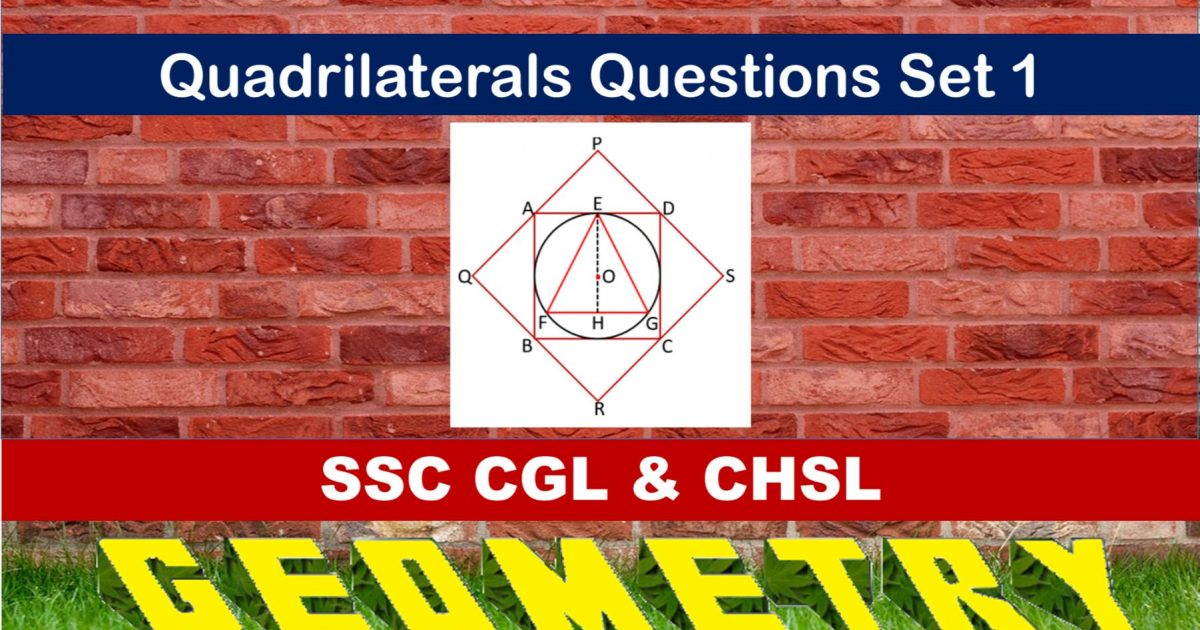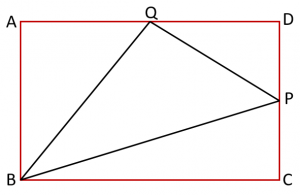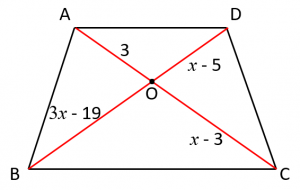Monday, September 27, 2021
Home > geometry > SSC CGL Geometry Quadrilateral Set 1

# SSC CGL Geometry Quadrilateral Set 1SSC CGL Geometry Quadrilateral Set 1 : Ques No 1

The length of the diagonal BD of the parallelogram ABCD is 18 cm. If P and Q are the centroid of ∆ABC and ∆ABC respectively, length of PQ is

Options:

A. 4 cm

B. 12 cm

C. 6 cm

D. 9 cm

Solution:

SSC CGL Geometry Quadrilateral Set 1 : Ques No 2

In rhombus ABCD, a straight line through C cuts extended AD at P and extended AD at P and extended AB at Q. If DP = (1/2)AB, then the ratio of BQ : AB is

Options:

A. 1 : 2

B. 2 : 1

C. 3 : 1

D. 1 : 1

Solution:

SSC CGL Geometry Quadrilateral Set 1 : Ques No 3

ABCD is a trapezium whose side AD is parallel to BC. Diagonals AC and BD intersect at O. If AO = 3, CO = x – 3, BO = 3x – 19 and DO = x – 5, the values of x will be

Options:

A. 7, 10

B. 7, 6

C. 12, 6

D. 8 : 9

Solution:

SSC CGL Geometry Quadrilateral Set 1 : Ques No 4

Inside a square ABCD, the triangle BCE is an equilateral triangle. If CE and BD intersect at O, then the value of the angle BOC is

Options:

A. 75 degrees

B. 60 degrees

C. 90 degrees

D. 120 degrees

Solution:

SSC CGL Geometry Quadrilateral Set 1 : Ques No 5

The side AB of a parallelogram ABCD is extended to E such that BE = AB and DE intersects BC at Q. Then the point Q divides BC in the ratio

Options:

A. 1 : 2

B. 2 : 1

C. 1 : 1

D. 2 : 3

Solution:

SSC CGL Geometry Quadrilateral Set 1 : Ques No 6

ABCD is a rhombus whose side AB = 4 cm and angle ABC = 120 degrees. Then the length of the diagonal BD is

Options:

A. 4 cm

B. 2 cm

C. 1 cm

D. 3 cm

Solution:SSC CGL Geometry Quadrilateral Set 1 : Ques No 7

In a rhombus ABCD, AB is produced to F and BA is produced to E such that AB = AE = BF. Then

Options:

A. ED ⊥ CF

B. ED > CF

C. ED2 + CF2 = EF2

D. ED || CF

Solution:

SSC CGL Geometry Quadrilateral Set 1 : Ques No 8

In a quadrilateral ABCD with unequal sides if the diagonals AC and BD intersect at right angles then

Options:

A. AB2 + BC2 = 2(CD2 + DA2)

B. AB2 + BC2 = CD2 + DA2

C. AB2 + CD2 = BC2 + DA2

D. AB2 + AD2 = BC2 + CD2

Solution:

SSC CGL Geometry Quadrilateral Set 1 : Ques No 9

If P and Q are two points on sides AB and AD of a parallelogram ABCD respectively and areas of ∆CPD = A1 and that of ∆BQC = A2, then

Options:

A. 2A1 = A2

B. A1 = A2

C. A1 = 2A2

D. 2A1 = 3A2

Solution:

SSC CGL Geometry Quadrilateral Set 1 : Ques No 10

In the following figure, a square ABCD is formed with its vertices as the mid-points of a larger square PQRS. A circle is inscribed in square ABCD and ∆EFG is an equilateral triangle inscribed in the circle. If the length of side of square PQRS is a, then the area of ∆EFG isOptions:

A. √3 a2/16

B. 3√3 a2/32

C. 5√3 a2/32

D. 5√3 a2/64

Solution:

SSC CGL Geometry Quadrilateral Set 1 : Ques No 11

In the figure below, P and Q are the mid-points of two sides CD and AD of a rectangle ABCD respectively. The ratio of the areas ∆BPQ and the rectangle ABCD isOptions:

A. 4 : 9

B. 5 : 8

C. 8 : 13

D. 3 : 8

Solution:

SSC CGL Geometry Quadrilateral Set 1 : Ques No 12

In a quadrilateral ABCD, ∠B = 90 degrees and AD2 = AB2 + BC2 + CD2, then ∠ACD is

Options:

A. 45 degrees

B. 60 degrees

C. 50 degrees

D. 90 degrees

Solution:

SSC CGL Geometry Quadrilateral Set 1 : Ques No 13

In the figure below, the lengths of the four sections of the two diagonals of a trapezium ABCD namely AO, BO, CO and DO are given. Then, find the possible values of x.Options:

A. 6, 8

B. 8, 9

C. 9, 7

D. 7, 8

Solution:

SSC CGL Geometry Quadrilateral Set 1 : Ques No 14

If the ratio of the angles of a quadrilateral is 2 : 7 : 2 : 7, then the shape of the quadrilateral is

Options:

A. is a rhombus

B. is a parallelogram

C. is a trapezium

D. cannot be identified

Solution:

SSC CGL Geometry Quadrilateral Set 1 : Ques No 15

If D, E and F are the mid-points of sides BC, CA and AB respectively of ∆ABC, then the ratio of the area of the parallelogram DEFB to the area of the trapezium CAFD is

Options:

A. 2 : 3

B. 3 : 4

C. 1 : 3

D. 1 : 2